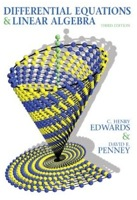### Differential Equations Math 2250-004 Spring 2018 Homework Page

Department of Mathematics
College of Science
University of UtahHomework assignments will be posted Wednesdays , a week before they are due - you will have a weekly quiz based on the homework problems, lab work, and the concepts they test, at the end of class on Wednesdays. A subset of the weekly homework problems are underlined and bold-face, and at the start of class on Wednesdays you will hand in carefully written up solutions to this subset of the homework. There will also be lab worksheets. These problems will be handed out in your lab meetings, and due at the start of lab the following week.
homeworkguidelines.pdf for written homework (thanks to Victor Camacho).

Due January 17
hw1.pdf
1.1: 6 = 2 points, 34 = 1 point
1.2: 6, 16 = 2 points each; 26abc = 1 point each, w1.1b = 2 points
1.3: w1.2a = 1 point, b = 2 points, c = 1 point.
1.4: 9, 12 = 2 points each.
Sections_1.1-1.3.pdf   and   Sections_1.3-1.5.pdf   of the text, for students who don't have the textbook yet.

Due January 24
hw2.pdf
EP3.7   supplementary section on RLC circuits, for two of the homework problems this week.
2.1-2.3texthw   text homework problems from 2.1-2.3 for people still waiting for their textbooks
1.4: 36 = 2 points, 46ab = 1 point each, 49 = 2 points
1.5: 7, 13, 14, 33, 36 = 2 points each
EP 3.7: 2 = 2 points
2.1: 6 = 2 points

Due January 31
hw3.pdf
2.1: 10, 12 = 2 points each; 23a = 1 point, 23b = 2 points, w3.1 = 2 points.
2.2: w3.2a = 2 points, b = 1 point
2.3: 2ab = 1 point each, 9, 13, 19 = 2 points each.

Due February 7
hw4.pdf
3.1: 6, 28 = 2 points each; 34 = 2 points (1/2 point each).
3.2: 8, 20 = 2 points each.
3.3: 20 = 2 points
w4.1, w4.2, w4.3a, w4.3b = 2 points each.

Due February 14
hw5.pdf
3.4: 10, 32ab = 2 points each
3.5: w5.1abcde = 2 points each, w5.2 = 4 points.

Due February 22 (at the start of your lab)
hw6.pdf
3.6: 6 = 2 points; w6.1abc = 2 points each, de = 1 point each.
4.1: 1, 26 = 2 points each; w6.2a = 1 point, bc = 2 points each, d = 1 point.

Due February 28
hw7.pdf
4.1: w7.1ab = 2 points each
4.2: 4, 6, 27 = 2 points each, w7.2 = 2 points
4.3: 8 = 2 points
4.4: 4, 6 = 2 points each; w7.3ab = 1 point each

Due March 7
hw8.pdf
4.4: w8.1abc = 2 points each, w8.2 = 2 points.
5.1: 6, 12, 14 = 2 points each; w8.3ab = 2 points each
5.2: 8 = 2 points.

Due March 14
hw9.pdf   The parts w9.3bcde are now optional - you will have a lab problem along these lines this week.
5.3: w9.1ab = 3 points each, w9.2 = 2 points.
5.4: 4, 6, 15, 17, 21 = 2 points each; w9.3a = 2 points.

Due March 28
hw10.pdf
5.5: 3, 31 = 2 points each. w10.1abcde = 2 points each.
5.6: 1, 7, 17 = 2 points each.

Due April 5 at the start of your lab
hw11.pdf
w12.1, w12.2, w12.3a, w12.4a, 10.2.20, w11.5, w11.6 = 2 points each. w11.7a = 3 points, w11.7b = 1 point; w11.8a = 2 points.

Due April 11
hw12.pdf
EP7.6
10.5: 31, w12.1, w12.2ab = 2 points each.
10.4: 36, 37 = 2 points each. w12.3 = 4 points extra credit.
EP7.6: 2 = 2 points
6.1: w12.4abc = 2 points each.

Due April 18
hw13.pdf